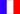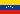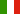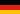# home | Archive | analysis | videos | data | weblognews in other languages:frespitade## Analysis of Venezuela's referendum counts

### By Jonathan Taylor

I was asked by Dr. Jennifer McCoy of the Carter Center, to look at the machine by machine counts of the recall election in Venezuela, specifically to see if there was any evidence of the opposition's claim of election fraud. The main issue I was asked to look at was the number of ties both for SI and NO at each mesa and determine if there were an excessive number of ties for SI.

In my analysis, I looked at several models for the count data using R, among them:

1. A model based on the empirical SI votes across all machines. R code
2. A Poisson model with one parameter shared by all machines. R code
3. A Poisson model with a parameter varying by mesa. R code

4. A multinomial model where the SI votes are redistributed across all machines within a mesa (p=(1/3,1/3,1/3) if there were three machines, p=(1/2,1/2) if there were two machines). R code
5. A parametric bootstrap model for the counts in each machine within each mesa, having conditionally independent Binomial counts within each machine with probability of success the observed proportion of SI votes within the mesa. R code
6. Another parametric bootstrap model, where the residuals were resampled assuming they were (conditionally) independent (given the counts). This was a mistake (see below). R code

Of these, the first two models are clearly unrealistic but were used for comparison. The next three are more realistic and give roughly the same results (see below). They also agree fairly well with simulation results of Avi Rubin at Johns Hopkins University. The multinomial model (the model with p=(1/3,1/3,1/3)) has already been analyzed by a statistician, Ellio Valladares, at the University of Virginia. The final model has a mistake in it, and, unfortunately, was the one whose results were reported in The Economist.

I have provided R code for the analyses, though some are slow to run, particularly the multinomial model. No real attempt has been made to write them more efficiently, rather they were written with the aim of being easy to read. To rerun the analyses in R, simply use "source", i.e. to rerun the parametric bootstrap model (R code) type the following at the R prompt

source('http://www-stat.stanford.edu/~jtaylo/venezeula/normal-resid-model.R')

## Correction to the results in The Economist

There was an error in the figures quoted by the Economist in an article written by Dr. McCoy. The figures were based on the above parametric bootstrap model, and the error was based on a mistake on my part.

Specifically, I fit a multivariate normal to the scaled residuals between the number of votes in a given machine and the total number of votes in the mesa (scaled by the square root of the total number of votes in each mesa). Unfortunately, in my first models, I made the significant error by ignoring the multivariate aspect of the residuals and generated uncorrelated residuals for the parametric bootstrap. Because these residuals should be negatively correlated, ignoring the correlation had the effect of making the simulated totals in each machine have less separation then they should have. This led to an inflated number of expected ties, hence the figure of 380, as quoted in The Economist is too high. Model 6. above is not exactly the same model that the 380 was based on, but is very similar: the model whose results are reported here is a parametric bootstrap (assuming the residuals were normally distributed given the total); the model reported in the Economist was based on a non-parametric bootstrap model.

## Results for SI

 Model E(Ties) SD(Ties) Z 1. 58 8 43 2. 320 18 4.6 3. 344 19 3.1 4. 348 19 2.9 5. 346 19 3.0 6. 377 19 1.3

Standard errors above are based on a Poisson approximation, as the number of ties is (under all of these models) the sum of many independent, rare counts. It is likely a slight underestimate of the true standard error.

## Results for NO

 Model E(Ties) SD(Ties) Z 1. 55 7 -34.5 2. 273 17 -2.3 3. 290 17 -1.2 4. 294 17 -1.0 5. 290 17 -1.2 6. 334 18 1.2

Standard errors above are based on a Poisson approximation, as the number of ties is (under all of these models) the sum of many independent, rare counts. It is likely a slight underestimate of the true standard error.

## Summary

It seems that an expected number of ties between 345 and 350 is reasonable, as it came out from many different models. Using the Poisson assumption to estimate the standard error, it seems then that the probability of observing 402 or more ties for SI is between 1 and 3 in 1000. While this probability is small, I do not feel that it should be interpreted as overwhelming evidence of fraud.